U.S. Department of Transportation
1200 New Jersey Avenue, SE
Washington, DC 20590
202-366-4000

Federal Highway Administration Research and Technology
Coordinating, Developing, and Delivering Highway Transportation InnovationsThis report is an archived publication and may contain dated technical, contact, and link information
 Federal Highway Administration > Publications > Research Publications > Geotechnicals > 03089 > Dynamic Bridge Substructure Evaluation and Monitoring
 Publication Number: FHWA-RD-03-089 Date: September 2005

# Dynamic Bridge Substructure Evaluation and Monitoring

## Chapter 6. Structural Modeling and Parameter Estimation

### Introduction

The overall objective of the analytic modeling in this project was to provide a means for interpretation of the field data based on the predictable dynamics of a structure. Finite element modeling provided an analytical basis for understanding the resonant frequencies and mode shapes observed in the field data. Parameter estimation provided a means for determining bridge foundation stiffness properties from the field data, which can then be used to determine bridge foundation type and condition. One of the prime purposes of the research was to evaluate the capabilities of using parameter estimation to determine bridge substructure conditions and possibly foundation type, such as shallow or deep foundation system.

3-D FEM models were formulated for each of the three tested bridges-Trinity River, Old Reliance, and Woodville Road. For the Trinity River Bridge, separate FEM models were generated for bents 2 and 12 because bent 2 had piles plus a strip footing and bent 12 had only piles. Of the other two bridges, only one bent was tested for each bridge; thus, only one FEM model per bridge was generated. The FEM models were generated using geometry from plan drawings and geometric field measurements. Construction material properties were taken as standard values except where the field data were available, such as for the elastic moduli of concrete and soil.

3-D FEM models revealed the key mode shapes and resonant frequencies associated with each of these structures. Because the field instrumentation was confined to the plane of the tested bent, it was simpler to model the tested bent as a 2-D structure, and then conduct a 2-D finite element analysis; however, it was necessary to lump the tributary mass of the superstructure (bridge deck and girders) on the cap beam of the bent. The 3-D FEM models provided a means for determining the magnitude of the lumped mass as a function for each mode of interest. The 2-D FEM models served as the basis for parameter estimation, described in detail later in this chapter. Because of issues related to the field data, 2-D finite element modeling was conducted for only the Trinity River Bridge, whose superstructure consisted of only a concrete slab.

### 3-D ANSYS Model

The 3-D FEM models were formulated for all three tested bridges using ANSYS®, a commercial FEM software. For each FEM model, the bridge foundation stiffness and mass were represented by soil-substructure super-elements KSSS and MSSS, each of which is a 6-by-6 matrix described in the equations in figures 87 and 89. The calculation of specific elements in the matrices depends on the nature of the bridge foundation- whether the bridge columns act as individual piles or are supported by either a spread footing or a pile cap supported by a pile group. The following paragraphs describe the features of the 3-D FEM model for each of the tested bridges.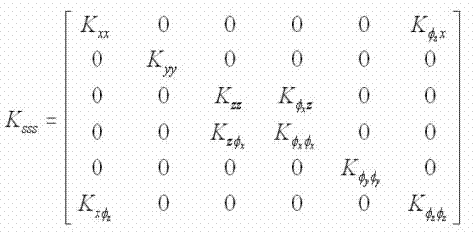Figure 87. Equation. KSSS.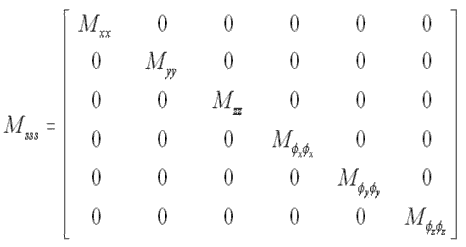Figure 88. Equation. MSSS.

#### Descriptions of 3-D FEM Modeling

The bents on the Trinity River, Old Reliance Road, and Woodville Road bridges tested in this research were all modeled with 3-D FEM techniques.

Trinity River Bridge (Bents 2 and 12)

For the Trinity River Bridge, shell elements were used to capture the out-of-plane bending of the bridge deck; beam elements were used to model the cap beam and concrete columns of the bent. Because the neutral axis of the bridge deck element is 0.508 m (20 inches) above the neutral axis of the cap beam, coupling was introduced numerically to model this connection. Because the bridge deck was supported simply by the bent, the corresponding bridge deck and bent elements were coupled in translation, but they were free to rotate with respect to each other. The cap beam has rigid connections with the concrete columns of the bent. The cross-sectional and material properties were calculated using standard strength of material techniques. The low-strain maximum Young's modulus for the concrete in the bent was computed from the ultrasonic pulse velocity field data and estimated values of Poisson's ratio and concrete density. The shear modulus of the soil was calculated from the SASW velocity data collected at the site and estimated values of Poisson's ratio and soil density. The bridge foundations were modeled using lumped stiffness (KSSS) and mass (MSSS) matrices, as explained by Santini.(134)

FEM models reflecting different locations of the Vibroseis truck were created for each tested bent of the Trinity River Relief Bridge. A lumped mass element was used to represent the mass of the Vibroseis truck. The truck mass was distributed as follows: 15,890 kg (35,000 lb) at the loading point; 2,724 kg (6,000 lb) at the position of the front wheels; and 3,178 kg (7,000 lb) at the position of the rear wheels. A 3-D FEM model for bent 2 consisted of 1,400 elements and 8,353 DOFs, while a 3-D FEM model for bent 12 consisted of 1,349 elements and 8,215 DOFs. Santini discusses in detail the calculations used to produce the 3-D FEM models for bents 2 and 12 of the Trinity River Bridge.(134)

Shell elements were used to model the bridge deck and girders because of the height-tothickness ratio of the girders-1.37 m-to-0.381 m (4.6 ft-to-1.25 ft). The use of the shell elements for the girders also provides a means for modeling the vertical offset between the bridge deck and the cap beam. The cap beam and columns, modeled as beam elements, were rigidly connected to each other. The cross-sectional and material properties were calculated using standard strength of material techniques. Santini made the detailed calculations for the elements in the lumped stiffness (KSSS) and mass (MSSS) matrices.(134) The resulting 3-D FEM model for the Old Reliance Road Bridge consisted of 4,639 elements and 29,298 DOFs; the 3-D FEM model for the Woodville Road Bridge consisted of 5,255 elements and 31,380 DOFs.

Only two loading locations of the Vibroseis truck were designated for these two bridges. Because of the large tributary mass of the bridge deck compared with the truck mass, the truck mass was ignored in the FEM modeling. Santini provides a detailed account of the calculations used to produce the 3-D FEM models for the Old Reliance Road and the Woodville Road bridges.(134)

#### Results of 3-D FEM Modeling

After each model was completed, a modal analysis was run in ANSYS, and subspace iteration was used. Only the first 60 modes were requested. The first 15 resonant frequencies for each model are shown in table 4, and Santini describes the mode shapes.(134)

Table 4. First 15 resonant frequencies in hertz for 3-D ANSYS FEM models.

1 11.06611.66010.54611.0332.07482.457
2 14.34514.81513.40613.6802.89103.470
3 14.81015.06613.67814.0723.36753.873
4 16.66816.14215.07414.6483.87304.353
5 18.74618.82216.28716.2874.21824.473
6 22.47023.60516.28816.2884.30355.018
7 23.58423.81116.28816.2884.63055.155
8 26.76725.59317.27617.3274.77635.656
9 27.29826.77120.35721.2845.92275.956
10 32.54231.55725.28623.5986.68926.703
11 33.00133.55826.04425.2926.73396.985
12 35.26635.23328.08325.5728.52837.136
13 37.05639.39128.09228.0838.94159.257
14 39.35239.58028.11628.09210.3359.752
15 40.26040.08630.80930.99911.07810.265

Trinity River Bridge Bent 12

Because the mass of the Vibroseis truck is part of the FEM model, the location of the truck affects the model; therefore, table 4 reports resonant frequencies from both side and center loading-the Vibroseis truck in different locations for each. These resonant frequencies range from 11 Hz to 40 Hz. Generally, the resonant frequencies excited by the center loading closely track those excited by the side loading. According to Santini, the mode shapes of the first 4 modes and 8 of the remaining 11 reported modes show motion only in the plane of the bent.(134) This behavior suggests that a 2-D FEM model would capture the significant behavior of the bent as measured in the field. Judging from the plotted mode shapes, the primary motion at the bridge foundations is vertical, which is the expected response with vertical excitation. Certain modes reveal horizontal motion, such as the eighth mode at 26.7 Hz for the side loading of bent 12. However, these modes are not revealed in the field data.

Trinity River Bridge Bent 2

For both side and center loading, the first 15 resonant frequencies ranged from 10.5 Hz to 30.8 Hz; therefore, one effect of the strip footing appears to be a lowering of the higher resonant frequencies. As with bent 12, the first four modes from both side and center loading show motion only in the plane of the bent; however, fewer of the remaining 11 reported modes show motion only in the plane of the bent, indicating that the strip footing appears to induce more out-of-plane motion. This behavior suggests that a 2-D FEM model may not capture the significant behavior of the bent in this frequency range. For the first four modes, the predominant motion at the bridge foundation is vertical.

Table 4 shows that the first 15 resonant frequencies ranged from 2.1 Hz to 11.1 Hz. Unlike the Trinity River Bridge, where mostly vertical motion occurred for the lower modes, the lower modes of the Old Reliance Road Bridge show considerable lateral movement both in and out of the plane of the bent. For example, the first and second modes are sideways in the plane of the bent, and the third and fourth modes combine this sideways movement with rocking in the direction of the roadway. This behavior difference may result from several factors-increased vertical stiffness of the bridge foundation used in the FEM model, exclusion of the truck mass, longer spans with different lengths leading to higher lateral frequencies, or a combination of these factors. Also, the height-to-width ratio for this bridge is greater than that for the Trinity River Bridge, making this bridge more prone to the observed motion.

Table 4 shows the first 15 resonant frequencies ranging between 2.5 Hz and 10.3 Hz, which is similar to that of the Old Reliance Road Bridge. Although this bridge has no skew, the observed modes show the motion both in and out of the plane of the bent. As with the Old Reliance Road, the predominant motion in the lower modes is horizontal. In fact, vertical motion begins to appear only in the eleventh and twelfth modes. The eleventh mode of this bridge is equivalent in motion to the first mode, and the twelfth mode is equivalent to the third mode under the center loading for bents 2 and 12 of the Trinity River Bridge.

### 2-D ANSYS Models

2-D FEM models serve as the baselines for the parameter estimation software. The parameter estimations were conducted only on bents 2 and 12 of the Trinity River Bridge. These estimations were conducted because of either the slightly poorer quality field data or the inability of the parameter estimation software to deal with variability in field data, or both.

In 2-D FEM models, the cap beam and columns of the bent, modeled with beam elements, were rigidly connected to each other. Because the bridge deck is not included in the 2-D FEM model, its mass and stiffness effects must be lumped onto the cap beam so that the resulting dynamic behavior of the bent is equivalent to what would be observed with the 3-D FEM model. However, the stiffness of the bridge deck does not appear to be significant in the lower modes of interest; thus, it is ignored here. Note that the absence of the third dimension causes certain modes representing out-of-plane motion in the 3-D FEM model not to appear in the 2-D FEM model. For each 2-D FEM model, the bridge foundation stiffness and mass were also represented by soil-substructure super-elements KSSS and MSSS, each of which is a 3-by-3 matrix, described in the equations in figures 89 and 90.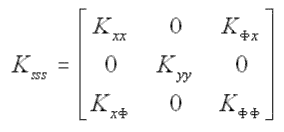Figure 89. Equation. KSSS(3 by 3 matrix).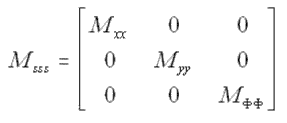Figure 90. Equation. MSSS (3 by 3 matrix).

The Rayleigh-Ritz method is used to determine the tributary deck mass to be lumped onto the cap beam. This energy-based method allows practitioners to select locations of the lumped masses and determine the equivalent masses that produce the same resonant frequencies as the structure with the distributed mass. In this project, the 3-D FEM models represent the structures with the distributed mass, and the 2-D FEM models have the equivalent lumped masses. Assuming that the tributary deck mass is M, the factor of alpha for each 2-D mode is determined so that when alpha M is placed on the cap beam, the resonant frequency of each 2-D mode equals that of the corresponding 3-D mode. The calculation of alpha is detailed in the following paragraphs.

#### Rayleigh-Ritz Method

The Rayleigh-Ritz method is an energy-based method to estimate the resonant frequency of a structure given the approximate mode shape. The maximum kinetic energy (KEmax) and the maximum potential or strain energy (SEmax) of a structure are calculated using the known mode shape or the estimated mode shape from the known static-deformed shape. For a structure with distributed mass and stiffness, KEmax and SEmax can be formulated as shown in the equations in figures 91 and 92, respectively, where Φn is the mode shape associated with the resonant frequency of ωn, and m(x) and EI(x) are the distributed mass and stiffness properties, respectively.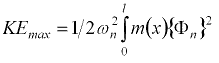Figure 91. Equation. KEmax (distributed properties).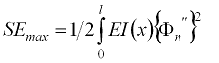Figure 92. Equation. SE max (distributed properties).

For a structure with lumped mass and distributed stiffness, KEmax and SEmax can be modified as in the equations in figures 93 and 94, respectively, where M is the number of lumped mass and Φi is the displacement at the location of the lumped mass at i, or (Mi). It should be mentioned that the equation in figure 92 is the same as the equation in figure 94, because both structures are assumed to have the same distributed stiffness properties, EI(x), and the same mode shapes, Φn.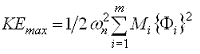Figure 93. Equation. KEmax (lumped properties).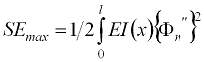Figure 94. Equation. SE max (lumped properties).

It is apparent that the effective amount of the lumped mass, M, can be found by equating the equations in figures 91 and 93, assuming that each lumped mass, Mi, has the same effective amount, M. Because the maximum kinetic energy, KEmax, and the maximum strain energy, SEmax, of a structure should be equal, the resonant frequency of Φn can be estimated by setting the equation in figure 91 equal to the equation in figure 92 or the equation in figure 93 equal to the equation in figure 94. The applications of the Rayleigh- Ritz method to both a simply supported and a cantilever beam demonstrated that the effective amount of the lumped mass depends on the structure type, the location of the lumped mass, and the specified mode shape.

#### Description of 2-D FEM Modeling

The 2-D FEM models for bents 2 and 12 of the Trinity River Bridge were created in both ANSYS and PARIS©, a static and dynamic PARameter Identification System program for a linear-elastic structure.1 The 2-D FEM models were created using the same cross section and material properties as in the 3-D ANSYS models except for the material densities of the beam elements. Taking into consideration the tributary mass of the bridge deck, the densities of the beam elements in the 2-D FEM models were multiplied by the alpha factors for each mode.

The initial 2-D FEM models using ANSYS were created assuming the tributary mass of the bridge deck from midspan to midspan. The resulting mode shapes and resonant frequencies from the initial 2-D FEM models were compared with those from the 3-D ANSYS models. Based on the Rayleigh-Ritz method, the resonant frequencies should be the same if the mode shapes from the two FEM ANSYS models are identical. Consequently, the alpha factors can be obtained easily by matching the resonant frequencies for the identical mode shapes from the 2-D and 3-D ANSYS models, as shown in the equation in figure 95.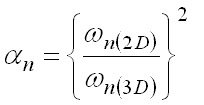Figure 95. Equation. α N.

It can be proven that the alpha factors obtained from the ratio of the resonant frequencies in the equation in figure 95 are the same as those from the procedure described earlier. After the alpha factors are determined for each mode, the 2-D FEM models using both ANSYS and PARIS are created with adjusted mass densities of the beam elements.

#### Results of 2-D FEM Modeling

The 2-D FEM models had 80 elements and 213 DOFs for bent 2, and 63 elements and 167 DOFs for bent 12. The elements and DOFs for bent 2 were higher because of the additional strip footing of bent 2. Each mode in each 2-D FEM model had a separate and unique alpha factor. Santini discusses the selected alpha factors and resonant frequencies based on the suitable field data.(134)

### Parameter Estimation with Paris Program

Parameter estimations were conducted using the PARIS system and software. Parameter estimation in this project is a procedure for determining unknown bridge foundation stiffness from selected frequency measurements and incomplete mode shapes. It represents an inverse problem, similar to those solved in geophysics for evaluating unknown geology and in pavements for backcalculating pavement layer moduli. Typical structural analysis is a forward-calculated problem, where the structure is considered known and the displacements, natural frequencies, and mode shapes are calculated for given loading conditions. Although the structure may be linear-that is, display linear elastic behavior and react with only small displacements-for the forward model, the inverse model of the same structure is algebraically nonlinear. Likewise, the results may be unique for the forward model, but the results may not be unique in solving the inverse problem. Following is a list of the basic steps of parameter estimation used in this project:

1. Identify the unknown structural parameters.
2. Develop a structural FEM model.
3. Assume the initial values for the unknown structural parameters.
4. Calculate natural frequencies and mode shapes using steps 2 and 3 or 7.
5. Develop an error function based on the calculated and measured data.
6. Minimize the error function for the unknown structural parameters.
7. Update the values for the unknown structural parameters.
8. Repeat steps 4 to 7 until convergence.

The solution for the updated values of the unknown structural parameters involves a set of linear equations defined by a sensitivity matrix. The condition of the sensitivity matrix determines the ability to solve for the unknown structural parameters. If the sensitivity matrix is singular or ill-conditioned, then a solution may not be found. At this point, the alternative for a given set of field measurements is to reduce the number of unknown structural parameters, which may lead to a solvable set of equations, but it involves assuming properties for certain structural parameters that are unknown. A second alternative is to anticipate the behavior of the sensitivity matrix before field measurements are made, which allows for the selection of field measurements that will lead to a better-conditioned set of equations.

As an inverse problem, small errors in the field measurements may be magnified in the solution process to produce much larger errors in the calculated unknown structural parameters; therefore, the influence of this measurement error must be evaluated and understood. Parameter estimation using a structural model before field data collection can be used to select the set of field measurements that are most error tolerant. Artificial errors may be added to the error-free simulated measurements from the structural FEM model, then PARIS is run with the contaminated simulated measurements to calculate the unknown structural parameters. By comparing the calculated values of the unknown structural parameters with those used in the structural FEM model, the sensitivities of the unknown structural parameters to different sets of simulated measurements are evaluated to determine the most error-tolerant sets of field measurements.

An underlying assumption in this project for parameter estimation is that structural parameters not identified as “unknown” actually are known. This is a grey area; some structural parameters may be better known than others, but they could differ significantly from actual values. For example, the foundation stiffness coefficients might be considered unknown and the column section properties known; however, the actual column section properties could be 25 to 50 percent different from the assumed values. The influence of this modeling error can be unacceptably magnified in the parameter estimation process. The influence of modeling error can be evaluated by simulation in a manner similar to the approach used for measurement error. If the influence of modeling error of a particular component is considered unacceptable, then that component may also be considered unknown. If it is found that the influence of modeling error is acceptably small, then the parameters estimation is successful.

#### Error Functions in PARIS

PARIS has both stiffness-based and flexibility-based modal error functions to detect deviations in each parameter from the original FEM model. This is done by using a selected number of resonant frequencies and associated mode shapes measured sparsely at certain DOFs. After this has been checked, the structural stiffness and mass parameters of the FEM model are updated at the element level to match the measured resonant frequencies and mode shapes. The stiffness-based modal error function was developed by Sanayei and Santini;(135) the flexibility-based modal error function was developed and programmed by Sanayei and Arya.(136)

Figure 96 is the equation for motion of an undamped free vibration. The equation can be partitioned, as shown in the equation in figure 97, for mode shapes at measured and unmeasured DOFs for each measured resonant frequency.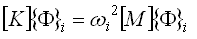Figure 96. Equation. Matrix K times vector Φ.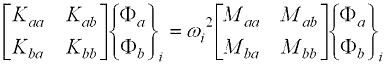Figure 97. Equation. Matrix K times vector Φ (partitioned).

[K] and [M] are the stiffness and mass matrices of the structure. Φi and ωi represent the mode shape at i and corresponding resonant frequency. The subscripts a and b denote the measured and unmeasured DOFs, respectively. By condensing out the unmeasured mode shapes Φb, the stiffness-based residual modal error function {es (p)}i can be expressed as the equation in figure 98, where (p) is the vector of the unknown parameters. The stiffness-based modal error function {Es (p)} can be derived by vertically appending the residual modal error function{es (p)}i. The resulting {Es(p)} is a 1 × a vector, where a is the number of the measured mode shapes.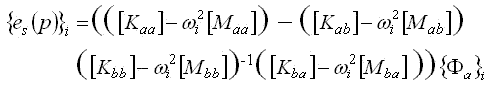Figure 98. Equation. Stiffness-based residual modal error function.

The equation in figure 99 is the flexibility-based residual modal error function {ef (p)} expressed in a form similar to the stiffness-based residual modal error function of the equation in figure 98.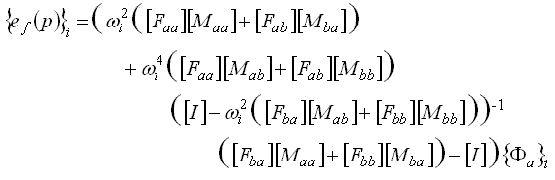Figure 99. Equation. Flexibility-based residual modal error function.

The flexibility-based error function {Ef (p)} also can be derived by vertically appending the residual modal error function {ef (p)}i. Both stiffness-based and flexibility-based residual error functions contain inverses of the stiffness matrices; therefore, they are algebraically nonlinear with respect to the unknown parameter vector (p).

#### Minimization of Modal Error Functions in PARIS

After it is established, the stiffness-based or flexibility-based error function {E (p)}, or both, can be approximated linearly, using Taylor Series expansion as shown in the equation in figure 100.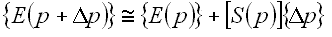Figure 100. Equation. Error function approximation.

The sensitivity matrix [S (p)] is the partial derivative of {E (p)}with respect to each of the unknown parameters. Furthermore, in the equation in figure 101, an objective function J is defined as the Euclidean norm of the modal error functions {E (p)}.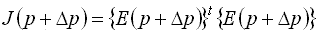Figure 101. Equation. Objective function J.

By minimizing the objective function J with respect to each unknown parameter, the change in each unknown parameter is determined by the Gauss-Newton formulation, as shown in the equation in figure 102.102. Equation. Change in parameter vector.

The updated values of the unknown parameters are calculated in the equation in figure 103, and they are used as the initial values for the next iteration until convergence is achieved.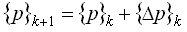Figure 103. Equation. Parameter iteration.

### Parameter Estimation Using Simulated Field Data

Before using the real field data in PARIS, parameter studies using the computersimulated field data were carried out for Trinity River Bridge bent 12 to evaluate the capacity of PARIS for bridge foundation stiffness estimation and the effects of measurement and modeling errors. There were four stages of parameter studies with simulated data:

1. Feasibility of bridge foundation stiffness estimations.
2. Feasibility of bridge foundation stiffness estimations adding beam and column properties as unknown parameters.
3. Effect of measurement errors.
4. Effect of modeling errors.

Based on the real field data from the final site visit, the first four modes at 11 receiver locations were extracted from the 2-D FEM PARIS model for bent 12. The first mode from the computer simulations was considered a pseudo-mode because the lateral stiffness of the bridge deck was not included in the 2-D FEM model; thus only the second, third, and fourth modes were used in the parameter studies. Also, only the stiffness-based modal error function was used in stages 1 and 2, while both stiffnessbased and flexibility-based modal error functions were used in stages 3 and 4.

#### Feasibility Study for Bridge Foundation Stiffness Estimation (Stage 1)

Table 5 summarizes the results of the parameter studies in stage 1. KSSS represents a fully populated bridge foundation stiffness matrix with six unknowns. K** represents the one with four nonzero unknowns, Kxx, Kyy, KΦΦ, and K, and K*** represents the one with three nonzero diagonal unknowns, Kxx, Kyy, and KΦΦ. “NA” in the column, “Number of Iterations,” means insufficient rank given the number of unknown parameters.

Table 5. Feasibility study for bridge foundation stiffness estimations.

Side 2,3,422664 × (Ksss)24244
Side 2,422444 × (Ksss)2420NA
Side 2,422444 × (K**)16164
Side 222224 × (K**)1612NA
Side 222224 × (K***)12124
Side 222224 × (Kyy)443
Center 2,3,422664 × (Ksss)24245
Center 2,422444 × (Ksss)2420NA
Center 2,422444 × (K**)16164
Center 222224 × (K**)1612NA
Center 222224 × (K***)12124
Center 222224 × (Kyy)443

The following conclusions can be drawn based purely on the ability to solve the equations, without regard to error in either the measurements or the modeling, or both:

• A minimum of three modes is required to calculate all six unknown parameters of bridge foundation stiffness.
• If only two modes are available, then two modes of the off-diagonal bridge foundation stiffness cannot be calculated.
• If only one mode is available, then only the diagonal bridge foundation stiffness can be evaluated.

#### Feasibility Study for Bridge Foundation Stiffness Estimation with Unknown Beam and Column Parameters (Stage 2)

The moments of inertia of the concrete beam and columns were identified as additional unknown parameters in stage 2 because of their uncertainties and significant effects in PARIS. Only the successful cases in table 5 were run with PARIS in stage 2. The parameter studies showed that an error of 10 percent in the moments of inertia of the concrete beam and columns would produce errors of up to 60 percent in the resulting bridge foundation stiffness. In addition, the uncertainties in the moments of inertia may result in either singular sensitivity matrices or dispersed cases; therefore, two additional unknown parameters were added in the PARIS runs to represent the moments of inertia of the beam cap (Ib) and columns (Ic), assuming all columns have the same moments of inertia. Table 6 summarizes the results of the parameter studies in stage 2, and it shows that all of the successful cases in table 5 also converged with unknown Ib and Ic in table 6.

Table 6. Bridge foundation stiffness estimations with unknown concrete beam and column moments of inertia.

Side 2,3,422664 × (Ksss),Ib,Ic26266
Side 2,422444 × (K**),Ib,Ic18186
Side 222224 × (K***),Ib,Ic14144
Side 222224 × (Kyy),Ib,Ic664
Center 2,3,422664 × (Ksss),Ib,Ic26266
Center 2,422444 × (K**),Ib,Ic18186
Center 222224 × (K***),Ib,Ic14145
Center 222224 × (Kyy),Ib,Ic664

#### Effect of Measurement Errors (Stage 3)

Effects of measurement errors on the PARIS runs have been studied by contaminating the computer-simulated resonant frequencies and corresponding mode shapes. PARIS has an option to assume the artificial errors to be uniform or normal distribution errors, using its user-defined internal functions. The artificial errors can be either proportional to the maximum values of the measurements or absolute values regardless of the magnitudes of the measurements. In this project, the corrupted computer-simulated field measurements with absolute uniform distributed errors were generated as shown in the equations in figures 104 and 105 to evaluate the error sensitivity of both stiffness-based and flexibility-based modal error functions.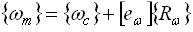Figure 104. Equation. Resonant frequency with artificial errors.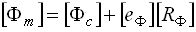Figure 105. Equation. Mode shape with artificial errors.

The terms {ωc} and [Φc] are, respectively, a pure computer-simulated resonant frequency vector and mode shape matrix from a 2-D PARIS model. [Rω] and [RΦ] are fully populated with uniform distributed random numbers between -1 and +1. The measurement errors with the prescribed level, [eω] and [eΦ], are multiplied by, respectively, the random numbers in [Rω] and [RΦ], and then added to the pure computersimulated field measurements {ωc} and [Φc].

Because the random numbers in [Rω] and [RΦ] are used, a Monte Carlo analysis is conducted to produce meaningful results. Although 100 to 1,000 observations normally are considered in a Monte Carlo analysis to gain a comprehensive understanding of the statistical properties of the output variables, only 10 observations are used for each of the prescribed measurement-error levels because of the relative small size and minimum complexity of 2-D PARIS models in this project.

Assuming that the percentage error for the jth unknown parameter estimated in the ith observation is PEji, the grand mean percentage error (GPE) and the grand standard deviation percentage error (GSD)(89) can be defined as in the equations in figures 106 and 107.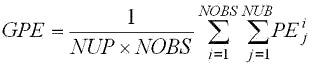Figure 106. Equation. Grand mean percentage error.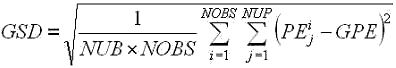Figure 107. Equation. Grand standard deviation percentage error.

NUP is the number of unknown parameters, and NOBS is the number of observations. Pji can be calculated as the deviation of the estimated value Pji from the true value Pjt, as in the equation in figure 108.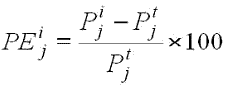Figure 108. Equation. Percentage error.

GPE and GSD can be used to compare the overall behaviors of the 2-D PARIS models to different levels of the input errors for all unknown parameters estimated in PARIS with several Monte Carlo experiments. When GSD is relatively small and GPE approaches zero for a prescribed measurement error level, the sensitivity of the 2-D PARIS models to the prescribed measurement error level can be determined. GPE will decrease as NOBS increases. In addition, the maximum value of PEji in each unknown parameter for any observation, MAX PEj, and the maximum value of PEji in any unknown parameter for any observation, MAX PEji, are examined to provide the vital insight into the unknown parameters that are overly sensitive to the measurement errors

Only one-mode cases in table 6 were considered in stage 3 because of the real field-data issues, which appeared to provide adequate data for one mode only. The objective of stage 3 was to ascertain which case was most measurement-error tolerant. Tables 7 and 8 summarize the results for the measurement-error analysis, showing that in general, very small measurement errors produced very large errors in the unknown parameters estimated in PARIS. For example, the most measurement-error-tolerant case was for center loading with Case Type II. For a 0.01 percent measurement error, the errors in the estimated Kyy ranged from 1.4 percent to 1.9 percent, and 4.2 percent and 4.8 percent for Ib and Ic, respectively. This represents an error magnification of 140 to 190 times for the bridge foundation stiffness estimations. Case Type I in tables 7 and 8 is the case with the unknown parameters of 4 × (K***), Ib, and Ic, while Case Type II is the case with the unknown parameters of 4 × (Kyy), Ib, and Ic.

Table 7. Effects of measurement errors (stiffness-based modal error function) using one-mode simulated field data.

Case TypeError (%)GPEGSDMAX PEijMAX PEj
IbIcK1xxK1yyK1ΦΦK2xxK2yyK2ΦΦK3xxK3yyK3ΦΦK4xxK4yyK4ΦΦ
Side
I0.001-7.5485.06653.01.68.34.81.05.83.32.314.03.14.412.04.76E39.8
I0.01-50.0869.01.2E418.0252.051.017.0554.041.023.0162.038.044.0264.031.01E4315.0
II0.001-0.93.959.01.84.9NA1.7NANA2.3NANA4.9NANA59.0NA
II0.01-16.043.0567.020.0107.0NA17.0NANA23.0NANA48.0NANA567NA
II0.1NANANANANANANANANANANANANANANANANA
Center
I0.001-0.66.639.010.01.78.03.136.06.62.123.07.41.818.05.13.539.0
I0.018.351.0208.017.0127.030.023.0208.056.020.0197.028.021.0129.031.031.0179.0
II0.001-0.62.97.81.87.8NA3.1NANA1.1NANA2.1NANA3.4NA
II0.01-9.729.083.018.083.0NA31.0NANA20.0NANA21.0NANA34.0NA
II0.1NANANANANANANANANANANANANANANANANA

Table 8. Effects of measurement errors (flexibility-based modal error function) using one-mode simulated field data.

Case TypeError (%)GPEGSDMAX PEijMAX PEj
IbIcK1xxK1yyK1ΦΦK2xxK2yyK2ΦΦK3xxK3yyK3ΦΦK4xxK4yyK4ΦΦ
Side
I0.0014.1619193.30.61.20.14.80.80.35.01.60.66.31.7919.09.2
I0.0112.01512,151.04.511.07.90.456.07.62.848.07.64.927.05.92E343.0
II0.0010.020.21.90.40.2NA0.06NANA0.2NANA0.5NANA1.9NA
II0.010.22.519.04.11.6NA0.6NANA2.3NANA5.1NANA18.0NA
II0.1-0.417160.030.017.0NA4.4NANA17.0NANA35NANA160.0NA
Center
I0.001NANANANANANANANANANANANANANANANANA
I0.01NANANANANANANANANANANANANANANANANA
II0.001-0.20.20.50.40.5NA0.2NANA0.2NANA0.1NANA0.2NA
II0.01-1.62.74.84.24.8NA1.6NANA1.9NANA1.4NANA1.5NA
II0.1-16.029100.030.099.0NA16.0NANA15.0NANA11.0NANA14.0NA

Review of MAX PEj reveals that the rotational stiffness, KΦΦ, is the most measurementerror- sensitive parameter because only vertical and horizontal, not rotational, mode shapes are measured in this project. Therefore, KΦΦ is eliminated from the list of unknown parameters. In addition, the horizontal stiffness, Kxx , and one of the vertical stiffnesses, K4yy, for the side-loading cases are considered as known parameters because of their high measurement-error sensitivities. The resulting most-measurement-errortolerance cases are 4 Kyy plus Ib and Ic for the center-loading cases, and 3 Kyy plus Ib and Ic for the side-loading cases.

#### Effect of Modeling Errors (Stage 4)

The measurement error analysis suggests that only vertical bridge foundation stiffness, Kyy, can be estimated using one-mode field data from vertical excitations used in this project. This means that the values of the other unknown bridge foundation stiffness variables must be assumed. Uncertainty of the assumed values causes modeling errors, and its effects on PARIS have been studied by manually changing the assumed values up to ±10 to 30 percent for each of the assumed parameters and their combinations. Only the two most measurement-error tolerance cases in stage 3 were used in the modeling-error analysis. Table 9 summarizes the results of the modeling-error analysis for the centerloading cases only, since the results for the side-loading cases are similar to those for the center-loading cases. Note that the elements with the initial value of zero in the soilsubstructure super-elements KSSS in the equation in figure 87, such as K, Kxz, and Kxy are also considered in the modeling-error analysis.

Table 9. Effects of modeling errors for the most measurement error tolerance case (center loading).

Modeling Error CombinationsModal Error FunctionMAX MPEj
IbIbK1yyK2yyK3yyK4yy
-20% in (Kxx, KΦΦ, K) and +20% in (K, Kxz)Stiffness-based9.285.00.41.03.14.5
Flexibility-based11.0296.035.025.033.056.0
-20% in (Kxx, KΦΦ, K, Kxz) and +20% in (K)Stiffness-based7.883.04.83.10.70.05
Flexibility-based21.0313.068.041.029.040.0
-20% in (Kxy), -15% in (Kxx, K), -10% in (KΦΦ), and +30% in (K)Stiffness-based7.580.04.83.10.71.3
Flexibility-based20.0319.070.042.030.041.0
-20% in (KΦΦ, K, Kxz) and +20% in (Kxx, K)Stiffness-based8.168.03.52.50.80.2
Flexibility-based14.071.013.013.02.915.0
-20% in (K1xx, K1ΦΦ, K1, K2xx, K2ΦΦ, K2, K2xz, K3xy, K4ΦΦ, K4, K4xz), -15% in (K3xx, K3), -10% in (K3ΦΦ), +20% in (K1, K1xz, K2, K4xx, K4), and +30% in (K3)Stiffness-based1.948.00.13.20.90.4
Flexibility-based59.0267.058.052.023.039.0

Review of table 9 shows that MAX PEj for all estimated Kyy, using the stiffness-based modalerror function, is less than 5 percent, which is small relative to the assumed modeling errors of up to 30 percent. Table 9 also shows that the flexibility-based modal error function is much more sensitive to the modeling errors than the stiffness-based modal error function. Therefore, because neither of them stands out as the optimum choice of both measurement errors and modeling errors, both stiffness-based and flexibility-based modal error functions were selected for analysis in the final PARIS runs with the real field data.

### Parameter Estimation Using Real Field Data

Parameter estimations using the real field data were performed for the two most error-tolerant cases on bents 2 and 12 of the Trinity River Bridge. Before doing this, it was necessary to match the experimental mode shapes and natural frequencies with those from 2-D FEM models. An exact match of the measured and analytical mode shapes is not likely, and it typically was not achieved. The possibility that the measured field mode shapes would be a combination of more than one natural mode severely affects the usefulness of these measurements in PARIS. Also, the measured mode shapes are assumed to be the 2-D response of the bridge pier only to the loading cases (center loading or side loading), but this is not necessarily the case.

The measured horizontal accelerations either were unreadable or unreliable because only vertical loading could be used in this project; therefore, only one up-down mode with little lateral motion was selected for the final PARIS runs, and only the vertical bridge foundation stiffness of each bent could be estimated. For bent 2, the up-down mode at the frequency of 14.6 Hz was selected for the center-loading case only. For bent 12, the up-down modes at the frequencies of 20.44 Hz and 20.40 Hz were selected for the side-loading and center-loading cases, respectively. Each of the measured mode shapes is assumed to contain only one mode, not a combination of two or more modes. The experimental mode results also indicated that the bridge foundations were asymmetrical.

#### Variations of Mode Shapes Extracted from Real Field Data

Variations of the mode shapes extracted from the real field data have been observed when using different bandwidths to process the same sets of the real field data. A bandwidth is defined as a frequency window in a transfer-function plot selected to extract a particular mode shape and its frequency. For a particular mode, different bandwidths produce the different mode shapes and frequencies with a maximum difference of up to 5 percent in terms of the resulting resonant frequency. All of the selected bandwidths were within the reasonable limits for a seasoned user of the PCMODAL curve-fitting software. The maximum difference of up to 5 percent certainly exceeds the most measurement-error tolerance of the analytical simulated field data study, which was usually less than 1 percent, as described in the following paragraphs on stage 3.

#### PARIS Runs Using Real Field Data for Bent 2

PARIS runs using only one suitable mode extracted from the real field data on bent 2 were conducted for the center-loading cases. This up-down mode has a resonant frequency of 14.6 Hz. Although both stiffness-based and flexibility-based modal-error functions were used in the PARIS runs, only the stiffness-based modal-error function produced acceptable values for the bridge foundation stiffness estimations for each of the four piles with a strip footing as shown in table 10. The initial values of the estimated vertical stiffness Kyy in PARIS runs are also presented in table 10, using analytical models of Novak et al.(137) and Poulos and Davis,(138) respectively. In fact, PARIS does not require accurate initial values for successful and meaningful parameter estimation. Even if the initial values are increased or decreased by 50 percent, the PARIS runs converged at exactly the same values, indicating the PARIS results were based on the global minimums instead of the local minimum of the stiffness-based modal error function.

Table 10. Initial and resulting Kyy in PARIS runs with real field data for bent 2.

K1yy × 106kg/m ( × 106lb/inch)K2yy × 106kg/m ( × 106lb/inch)K3yy × 106kg/m ( × 106lb/inch)K4yy × 106kg/m ( × 106lb/inch)
Novak model 89.3 (5.0)89.3 (5.0)89.3 (5.0)89.3 (5.0)
Poulos and Davis model 10.7 (0.6)10.7 (0.6)10.7 (0.6)10.7 (0.6)
Note: Reference Novak et al.(137) and Poulos and Davis.(138)

The resulting vertical Kyy were used in the 2-D ANSYS model for bent 2 to compare the resulting mode shapes and frequencies with the ones extracted from the real field data. As shown in table 11, the resonant frequencies from the 2-D ANSYS models (11.3 Hz and 14.7 Hz) do not match the resonant frequency from the real field data (20.0 Hz). This indicates that the resulting Kyy from the PARIS runs are not real, and the parameter estimation with PARIS using the real field data was unsuccessful for the center-loading cases of bent 2 (piles plus footing).

Table 11. Resonant frequencies from 2-D ANSYS models and real field data for the centerloading cases of bent 2 with an alpha of 1.056.

Mode Number2-D ANSYS Model with the Theoretical Kyy2-D ANSYS Model with the Resulting Kyy from PARIS RunsReal Field Data
1 3.943.98NA
2 11.314.720.0
3 13.720.4NA
4 25.929.2NA

#### PARIS Runs Using Real Field Data for Bent 12

For bent 12, PARIS runs using the real field data were carried out for both the center- and sideloading cases. The up-down vertical modes at the frequencies of 20.44 Hz and 20.40 Hz were extracted from the real field data for the side- and center-loading cases of bent 12, respectively. Like the case with bent 2, even though both stiffness- and flexibility-based modal error functions were run with PARIS, only the stiffness-based modal error function produced acceptable values for the bridge foundation stiffness estimations, as shown in table 12.

Table 12. Initial and resulting Kyy in PARIS runs with real field data for bent 12.

K1yy ×  106kg/m ( ×  106lb/inch)K2yy ×  106kg/m ( ×  106lb/inch)K3yy ×  106kg/m ( × 106lb/inch)K4yy × 106kg/m ( × 106lb/inch)
Novak model 89.3 (5.0)89.3 (5.0)89.3 (5.0)89.3 (5.0)
Poulos and Davis model 10.7 (0.6)10.7 (0.6)10.7 (0.6)10.7 (0.6)
Note: Reference Novak et al.(137) and Poulos and Davis.(138)

a K4yy must be assumed as known, denoted by “K,” to achieve reasonable convergence.

Just as for bent 2, the resulting Kyy were used in the 2-D ANSYS model for bent 12 to compare the mode shapes and frequencies with the ones extracted from the real field data. As shown in table 13, the resonant frequencies for the side-loading cases are relatively close for the visually matching mode shapes from the 2-D ANSYS models (14.5 Hz and 18.5 Hz) and the real field data (20.4 Hz); however, table 14 shows the resonant frequencies for the center-loading cases are not close for those from the 2-D ANSYS models (11.8 Hz and 13.8 Hz) and the real field data (20.0 Hz). The results in table 14 indicate that the Kyy vertical stiffness values for the four pile foundations from PARIS runs are not real for the center-loading of bent 12.

Table 13. Resonant frequencies from 2-D ANSYS models and real field data for the side-loading cases of bent 12 with an alpha of 1.035.

Mode Number2-D ANSYS Model with the Theoretical Kyy2-D ANSYS Model with the Resulting Kyy from PARIS RunsReal Field Data
1 3.944.03NA
2 11.216.014.8
3 14.518.520.4
4 21.626.227.7

Table 14. Resonant frequencies from 2-D ANSYS models and real field data for the centerloading cases of bent 12 with an alpha of 1.055.

Mode Number2-D ANSYS Model with the Theoretical Kyy2-D ANSYS Model with the Resulting Kyy from PARIS RunsReal Field Data
13.924.0115.0
211.813.820.0
314.518.527.4
419.223.3NA

1 PARIS, Parameters Identification Systems, is a copyrighted software developed by Professor Masoud Sanayei, Ph.D. and Tufts University, Department of Civil and Environmental Engineering, Medford, MA.